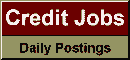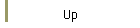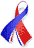DefaultRisk.com the web's biggest credit risk modeling resource.doi> search: A or B Export citation to:- HTML- Text (plain)- BibTeX- RIS- ReDIF

A Geometric Representation of the Frisch-Waugh-Lovell Theorem

by Walter Sosa Escudero of the Universidad Nacional de La Plata

March 21, 2001

Introduction: Even though the result recently referred to as the 'Frisch-Waugh-Lovell theorem' (FWL theorem, henceforth) has been around for a long time, it is relatively recently that it has been widely used by econometricians as a powerful pedagogical tool to express in a simple and intuitive way many results that often rely on tedious and seldom intuitive algebraic steps, which are also notationally cumbersome.

Even though a proof of the FWL theorem can be based entirely on standard algebraic results, the main reason of its increasing popularity is its strong geometric appeal. Recent texts and articles provide a mix between algebraic proofs and geometrical illustrations of the theorem, but none of them presents a fully geometrical proof of the result. The goal of this note is very modest: it extends the standard geometrical representations of the theorem to actually prove it based on geometrical arguments, which should, hopefully, provide a richer understanding of the scope of the theorem.

Books Referenced in this paper:  (what is this?)